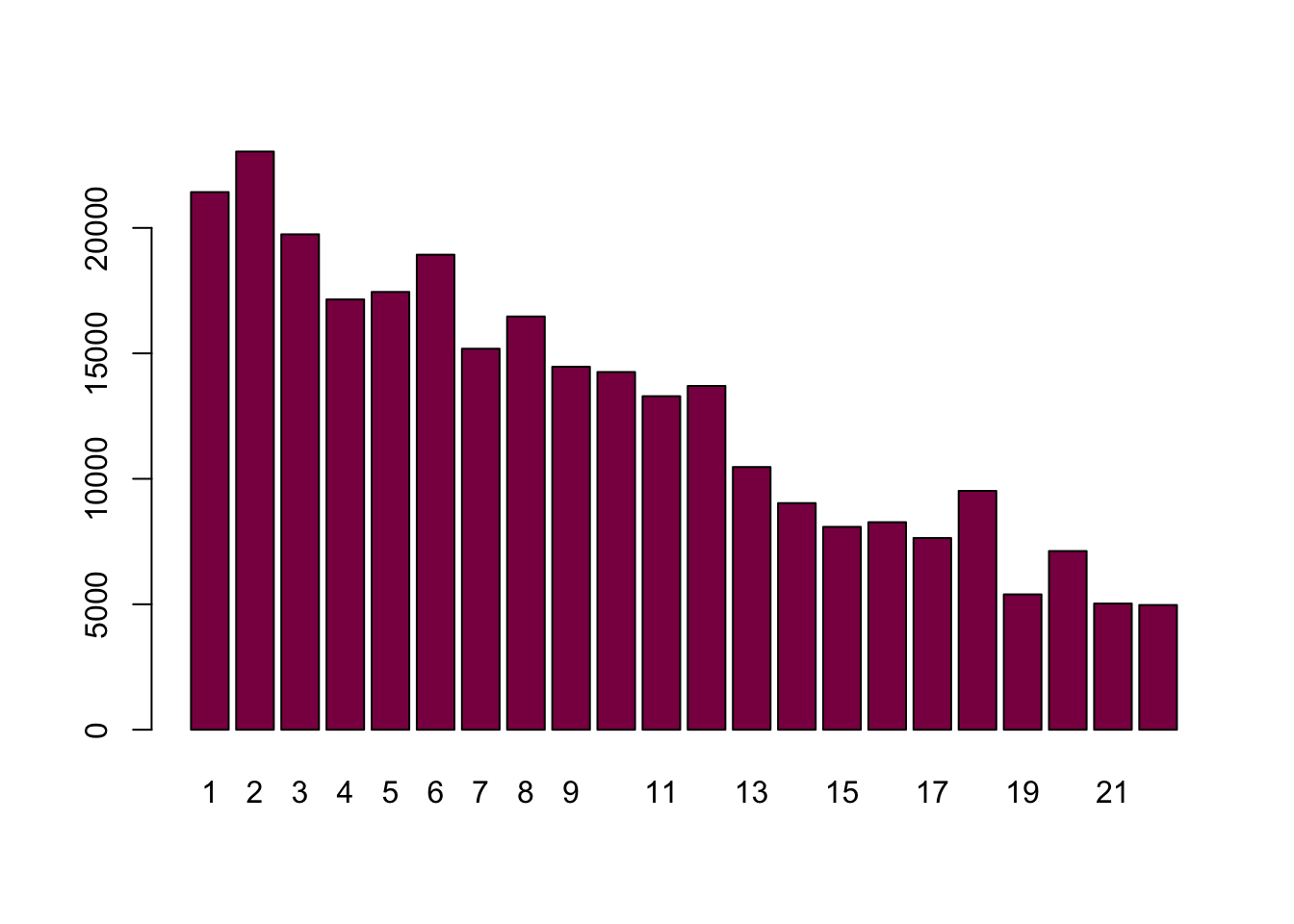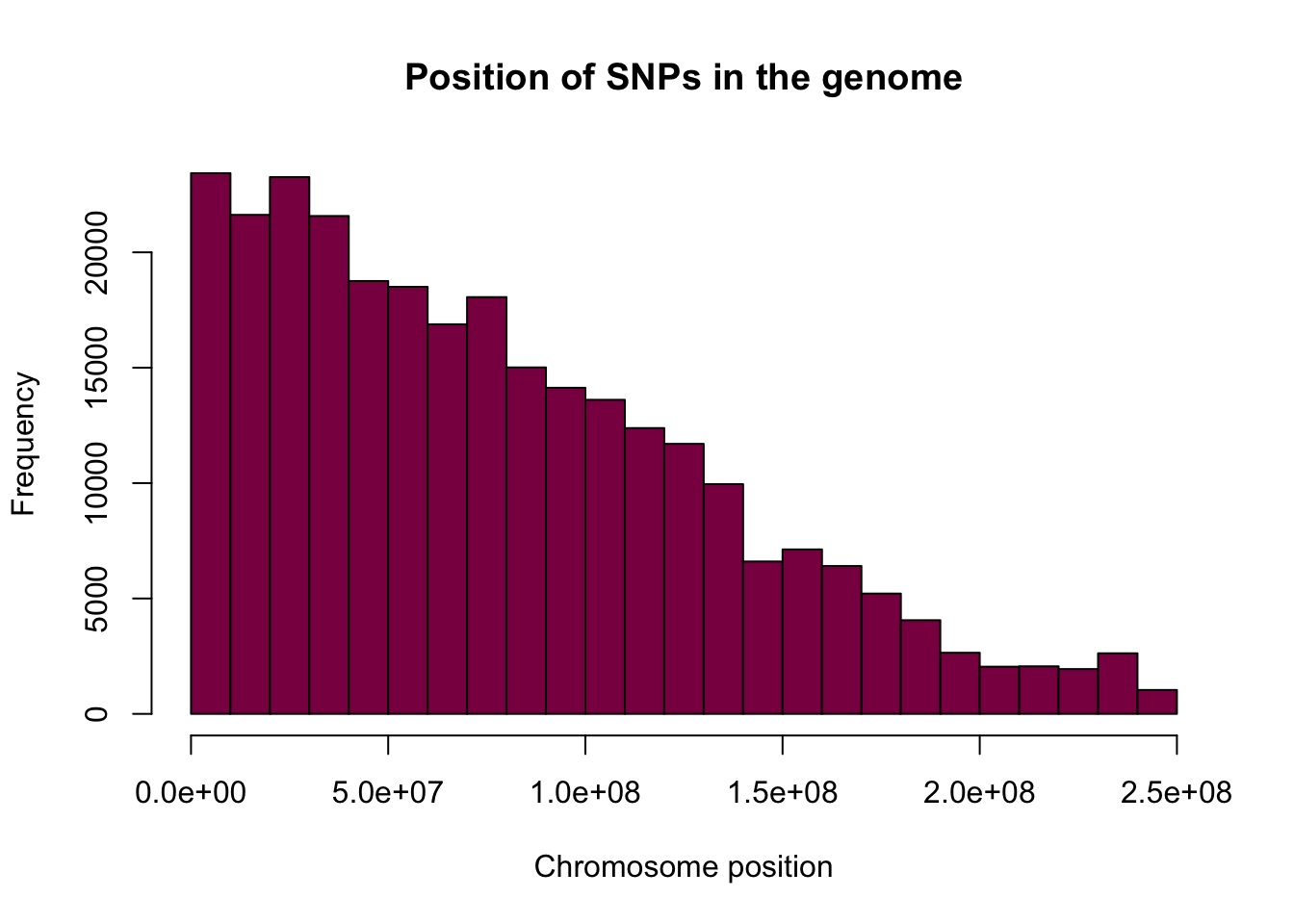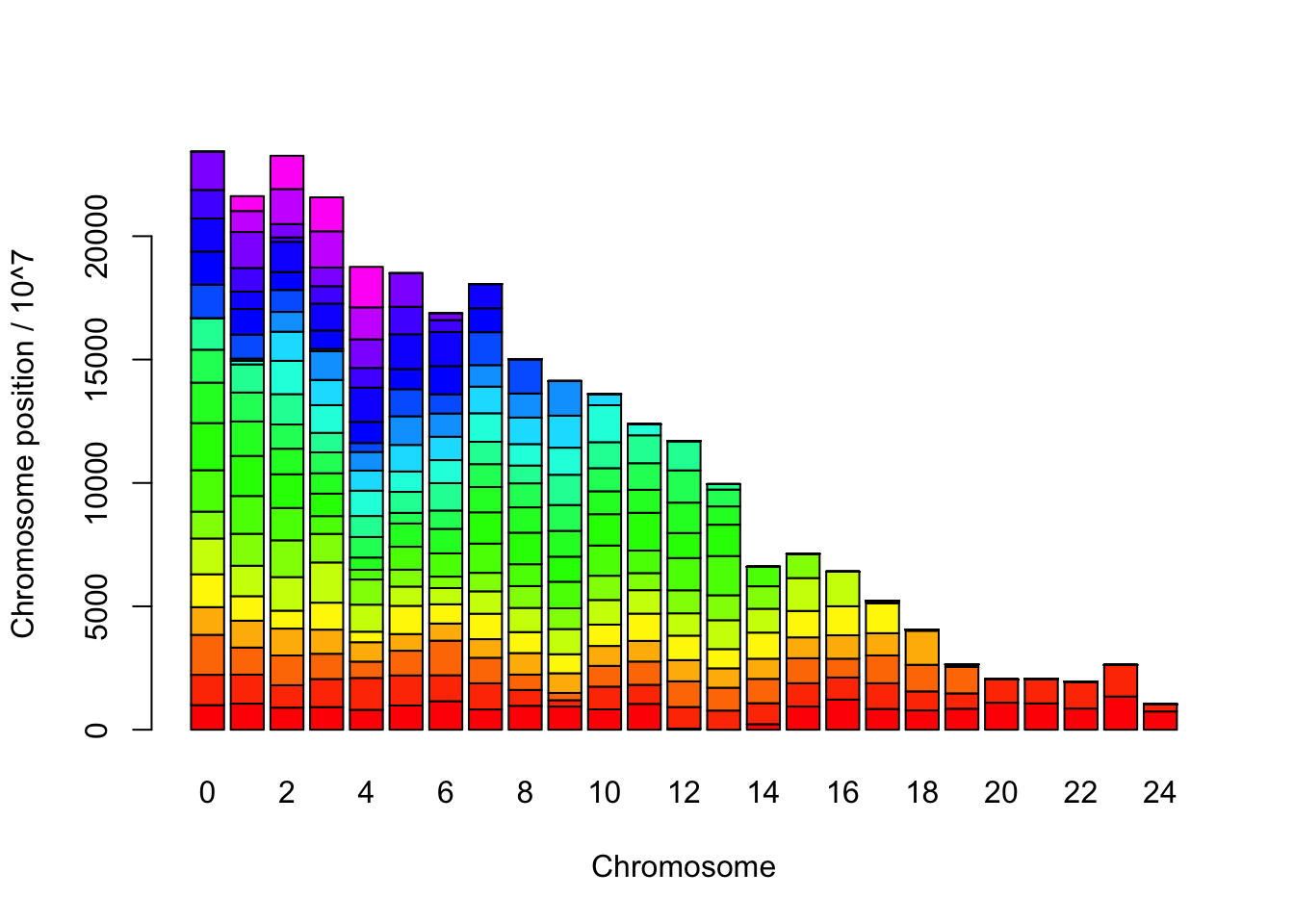### Learning goals

#### By the end of this session, you should be able to…

• calculate odds ratios by hand and associated values (confidence intervals etc.)
• visualize odds ratios
• identify extreme odds ratios
• calculate \(chi^2\) statitics by hand and use \(chi^2\) distribution to get a p-value
• simulate a null distribution to get a p-value
• use R’s \(chi^2\) test function to get a p-value
• use R’s Fisher’s exact test to get a p-value
• compare the results
• select a method and test all 280.000 SNPs
• identify interesting patterns
• explain the pattern by biomedical knowledge
``````library(tidyverse)
library(microbenchmark)``````

### GWAS project

First, we read the data and see what kind of variables we have.

``````snpdata = readRDS(file = "gwas_allele_counts.julia.2008.rds")
``````## # A tibble: 6 x 5
##   rsid       chromosome chromosome_posit~ genome_position contingency_tab~
##   <chr>           <int>             <int>           <dbl> <list>
## 1 rs3934834           1            995669          995669 <dbl [2 x 2]>
## 2 rs3737728           1           1011278         1011278 <dbl [2 x 2]>
## 3 rs6687776           1           1020428         1020428 <dbl [2 x 2]>
## 4 rs9651273           1           1021403         1021403 <dbl [2 x 2]>
## 5 rs4970405           1           1038818         1038818 <dbl [2 x 2]>
## 6 rs12726255          1           1039813         1039813 <dbl [2 x 2]>``````

This dataset is described in the paper “Genome-wide association study of rheumatoid arthritis in the Spanish population: KLF12 as a risk locus for rheumatoid arthritis susceptibility” by Julia et al.

The first three columns are easy:

rsid = SNP id
chromosome = the name of the chromosome
chromosome_position = the position of the SNP within the chromosome

We have taken all the chromosome and glues them together from chromosome 1…chromosome 22, then we made a new coordinate system starting from 1 (chromosome 1) and ending in position ~3 gigabases (chromosome 22). This position is calculated in the column called “genome_position”. Finally we have counted the number of alleles in cases and controls and put this contingency table into the variable “contingency_table”.

### Looking at the data

#### Count the number of SNPs per chromosome.

``table(snpdata\$chromosome)``
``````##
##     1     2     3     4     5     6     7     8     9    10    11    12
## 21427 23044 19745 17149 17448 18932 15185 16470 14467 14256 13293 13701
##    13    14    15    16    17    18    19    20    21    22
## 10470  9032  8085  8271  7639  9518  5394  7121  5029  4970``````

#### Plot the number of SNPs per chromosome.

``barplot(table(snpdata\$chromosome), col = "deeppink4")``We can see that the number of SNPs shows a decreasing tendency - this is likely due to the fact that the chromosomes have different lengths, and the longer the chromosome is, the more likely it is to have more SNPs on it.

Now we will look at the position of SNPs in the genome in more detail.

``````hist(snpdata\$chromosome_position, col = "deeppink4",
xlab = "Chromosome position",
main="Position of SNPs in the genome") ``````This tendency can also be explained by the chromosome size. All chromosomes are at least a few million bp long, but only the longest ones stretch to, say, \(1.5*10^8\) bps and beyond.

To illustrate this, let’s make a table of the chromosome positions per chromosome and make a plot.

``````x = table(snpdata\$chromosome, snpdata\$chromosome_position%/%10^7)
barplot(x, col=rainbow(25), xlab="Chromosome", ylab="Chromosome position / 10^7") ``````#### Make a histogram of the genome_position. First calculate the number of breaks needed so each bin is ~10 million bp, then make the histogram using that number of breaks.

``````nbreaks = round(max(snpdata\$genome_position) / 10^7)
hist(snpdata\$genome_position, col="deeppink4", breaks = nbreaks, border = NA,
xlab = "Genome position", main = "Histogram of genome position")``````